Next: Dirac Equation Up: Klein-Gordon Equation Previous: Summary

# Problems

1. Show that in the nonrelativistic limit, the free-particle Klein-Gordon equation becomes the free-particle Schrödinger equation.

2. The Lorentz-invariant step function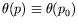is defined by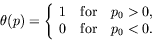It is said that this step function is Lorentz invariant because it only distinguishes between the past and the future, which is a Lorentz invariant concept. Show that this step function is Lorentz invariant.

Hint: I believe this is true only if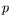is restricted to be a time-like vector.

3. Use explicit plane-wave solutions,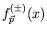, to establish the following normalization and orthogonality relationships: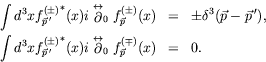4. Derive the conserved current for a scalar field interacting with an electromagnetic field with minimal coupling.

5. Show that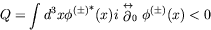is satisfied for a solution to the Klein-Gordon equation,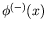, that is a superposition of negative energy plane-wave solutions.

6. Show that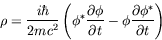reduces to the proper nonrelativistic expression in the nonrelativistic limit.

7. The positive and negative energy two-component solutions of the Klein-Gordon equation in the Schrödinger form were define as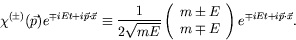Show that the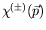are orthonormalized.

8. Show that in the nonrelativistic limit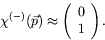9. By completeness, any wavepacket can be expanded in terms of a linear combination of positive and negative energy solutions: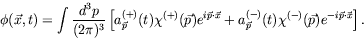Theare defined asand the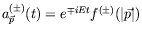, where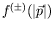are general scalar functions of the magnitude of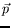. Derive the normalization requirement for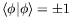.

10. Invertto obtain experessions for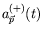and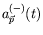.

11. Derive the expectation value of the position operator for a wavepacket that is a mixture of positive and negative energy components: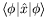. Show that your result contains a piece that represents the expect uniform velocity motion of the wavepacket and a piece that represents a rapid wiggling (Zitterbewegung) of the position of the particle about its central location, due to the interference of positive and negative energy components.

12. Solve the coulomb potential problem. In lectures we obtained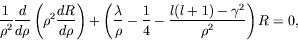where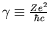,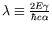,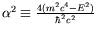, and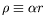.

Look for solutions that are finite at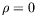and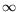and show that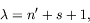where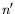is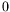or a positive integer and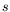is the non-negative solution of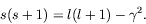Show the energy can be expanded in powers of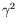and to order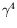is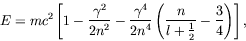where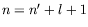is the total quantum number and can take on positive integer values. Identify the rest energy, the energy in the nonrelativistic theory and the fine-structure energy. Calculate the spread of the fine-structure levels for a given. (Note: they are much larger than obeserved experimentally in the hydrogen spectrum.)

13. The homogeneous Green function can be written as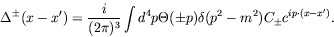Show that without loss of generality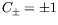.

14. Show that
1.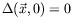,
2.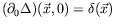,
3.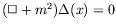,
4.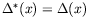,
5.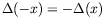,
6.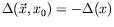,
7.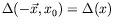,
8.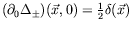.

15. Show that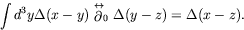16. Prove that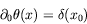and thus show that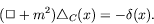17. Find the solution of the Klein-Gordon equation for the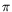-meson in a Coulomb potential and discusss the energy eigenvalues. The pion has a mass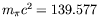MeV and spin 0.

18. Starting with a positive energy wavepacket only, show that there is a minimum width to the wavepacket.

19. Sarting with a positive energy wavepacket only, show that they can not be localized within a distance smaller than the Compton wavelength.

20. We have seen that the energy levels of a mesonic atom are given by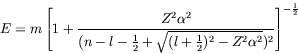1. Show that the ground state energy for any mesonic atom heavier than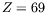is complex. Explain what this complex energy means.

2. Mesonic atoms have been well studied at places like Los Alamos and it has been found that the ground states of atoms as heavy as lead (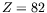) or uranium (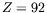) are quite stable. How do you reconcile this fact with the result obtained above? Be as quantitative as you can.

21. A rapidly varying electric field can lead to the creation of particle-antiparticle pairs. Calculate to lowest order in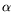the probability per unit volume per unit time of producing such pairs in the presence of an external electric field.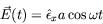and show that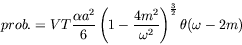Suggestion: Use as an interaction potential the usual form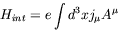where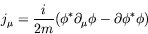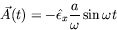Utilize normalized plane waves solutions of the Klein-Gordon equation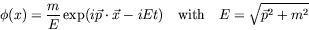and simple first order perturbation theory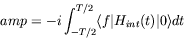22. Consider a free charge Klein-Gordon particle of mass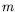and charge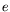immersed in a uniform magnetic field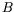in the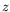-direction. Using the gauge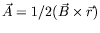show that motion is quantized with energy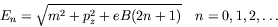23. Develop the wave equation for the nonrelativistic Hamiltonian developed from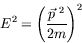and show that you get positive- and negative-energy solutions.

24. Show that the Schrödinger equation is not Lorentz invariant.Next: Dirac Equation Up: Klein-Gordon Equation Previous: Summary
Douglas M. Gingrich (gingrich@ ualberta.ca)
2004-03-18